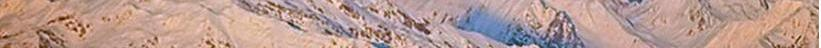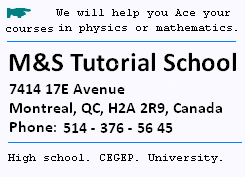Contents

© The scientific sentence. 2010# Definitions

### space time:

```spacetime is the frane where an event occurs. That is where
the position of a point is given by its coordinates in
space and time. t

```

### event:

```An event is an occurrence localized in space and time.

```

### frame of reference:

```A frame of reference refers to a coordinate system
within we make measurements.

```

### inertial frame:

```an inertial frame of reference, or Galilean reference frame,
is a frame of reference that is at rest or in rectilinear motion at
constant velocity with respect to another frame, then not accelerated.
In this frame, time and space are homogeneous (the same)
and isotropic (uniform in all directions).
Measurements in one inertial frame can be converted to
measurements in another inertial frame , by Galilean transformation
in Newtonian physics and the Lorentz transformation in special
relativity.

```

### observer:

```The term "observer" refers to hwo is making local measurements

```

### proper length:

```The proper length of an object is the length of this object
as measured by an observer which is at rest relative to the
object.

```

### proper length between two events:

```The proper length between two space-separated events is
the distance between these two events when they are
simultaneous.

In any inertial frame of reference, the proper
length L is:
L = [Δx2 + Δy2 + Δz2 - c2Δt2]1/2
Where:
-- Δx,  Δy,  and Δz are the difference of spacial coordinates
of the two events.
-- Δt is the difference in the temporal coordinates of the two events,
-- c is the speed of light.

```

### simultaneity::

```Simultaneity is the fact that two events occur at the same time,
unlike of absolute. Simultaneity  depends on the observer's
reference frame.

```

### Lorentz invariant :

```In a Euclidean space, the separation between two points
is the the distance between the two points. this distance is
spatial and positive. In spacetime, the separation between
two events is the interval  between the two events, that
takes into account both the spatial and tempora separation
between the events. The spacetime interval is:

s 2  = r2  - c2 t2

```

### priciples of relativity:

```-- The laws of Physics take the same form in all inertial frames
of reference.
-- The speed of light in vacuum is the same in all inertial frames
of reference. It is independent of the motion of the source or
the motion of the receiver.

```

 chimie labs | Physics and Measurements | Probability & Statistics | Combinatorics - Probability | Chimie | Optics | contact |